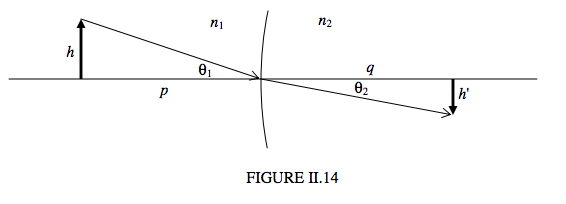Skip to main content
$$\require{cancel}$$

# 2.9: Derivation of Magnification

•• Contributed by Jeremy Tatum
• Emeritus Professor (Physics & Astronomy) at University of Victoria

Figure II.14 shows an optical element separating media of indices $$n_1$$ and $$n_2$$. I have drawn the element as an interface, though it could equally well be a lens (or, if I were to fold the drawing, a mirror). An image of height $$h'$$ is formed at a distance $$q$$ of an object of height $$h$$ at a distance $$p$$.Assuming, as ever, that angles are small, we have

$\text{magnification} = \dfrac{\theta_2q}{\theta_1p}.$

But Snell’s law, for small angles, is $$n_1\theta_1 = n_2\theta_2$$ , and therefore

$\text{magnification} = \dfrac{n_1q}{n_2p} = \frac{C_1}{C_2}. \label{eq:2.9.1}$